# Euler characteristic of covering space is product of degree of covering and Euler characteristic of base

## Statement

Suppose$E$ and$B$ are both topological spaces, with$B$ a path-connected space, and$p:E \to B$ is a covering map (surjective) having finite degree. If$E$ and$B$ both have finitely generated homology, then we have the following relation: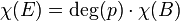$\chi(E) = \operatorname{deg}(p) \cdot \chi(B)$

where:

•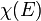$\chi(E)$ is the Euler characteristic (?) of$E$.
•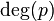$\operatorname{deg}(p)$ is the degree of the covering map$p$, i.e., the size of any of the fibers. The fibers all have the same size because the base space is path-connected and the map is a covering map.
•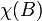$\chi(B)$ is the Euler characteristic (?) of$B$.

## Particular cases

The Poincare polynomial of a topological space is the ordinary generating function of the Betti numbers, and evaluating this polynomial at$-1$ gives the Euler characteristic.

Degree of covering Parameter Base space Homology information Poincare polynomial Euler characteristic Covering space Homology information Poincare polynomial Euler characteristic
2 Odd nonnegative integer$n$ real projective space$\R\mathbb{P}^n$ link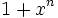$1 + x^n$ 0 sphere$S^n$ link$1 + x^n$ 0
2 Even nonnegative integer$n$ real projective space$\R\mathbb{P}^n$ link$1$ 1 sphere$S^n$ link$1 + x^n$ 2
2 -- Klein bottle link$1 + x$ 0 2-torus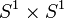$S^1 \times S^1$ link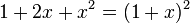$1 + 2x + x^2 = (1 + x)^2$ 0$m$ -- circle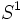$S^1$ link$1 + x$ 0 circle$S^1$ (via$m^{th}$ power map in$\mathbb{C}$) link$1 + x$ 0